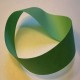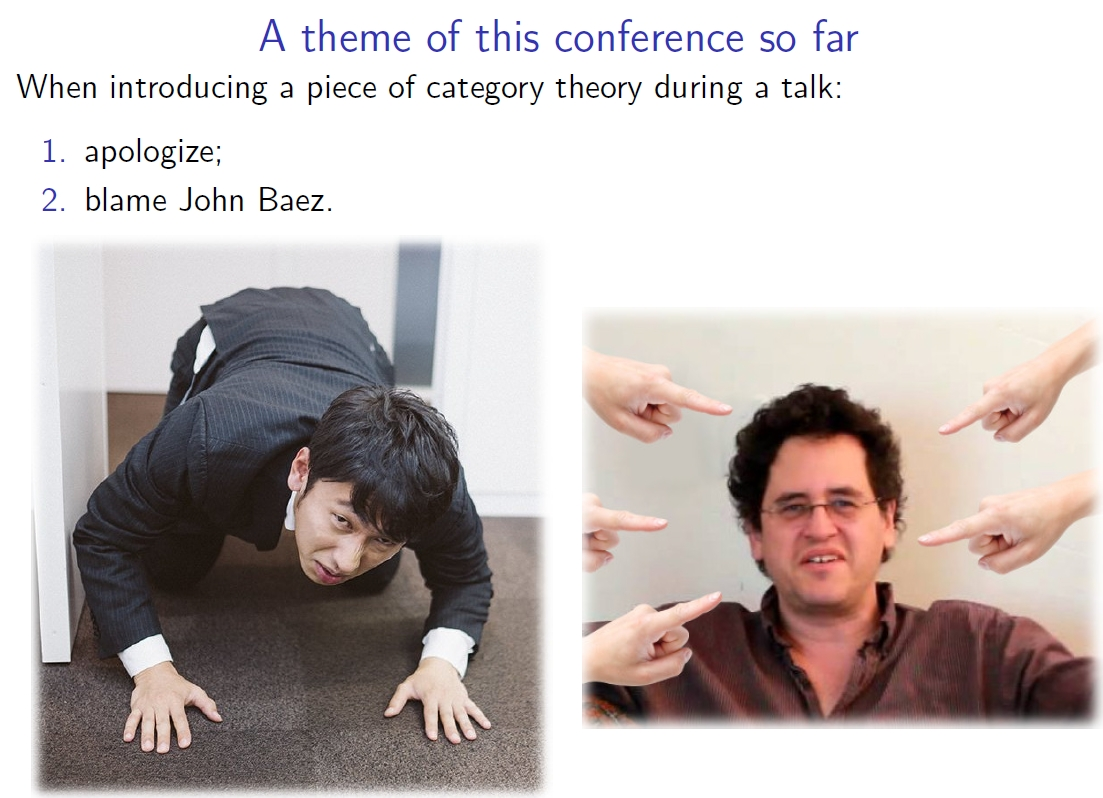## The Rise and Spread of Algebraic TopologyPeople have been using algebraic topology in data analysis these days, so we’re starting to see conferences like this:

Applied Algebraic Topology 2017, August 8-12, 2017, Hokkaido University, Sapporo, Japan.

I’m giving the first talk at this one. I’ve done a lot of work on applied category theory, but only a bit on on applied algebraic topology. It was tempting to smuggle in some categories, operads and props under the guise of algebraic topology. But decided it would be more useful, as a kind of prelude to the conference, to say a bit about the overall history of algebraic topology, and its inner logic: how it was inevitably driven to categories, and then 2-categories, and then ∞-categories.

This may be the least ‘applied’ of all the talks at this conference, but I’m hoping it will at least trigger some interesting thoughts. We don’t want the ‘applied’ folks to forget the grand view that algebraic topology has to offer!

Here are my talk slides:

Abstract. As algebraic topology becomes more important in applied mathematics it is worth looking back to see how this subject has changed our outlook on mathematics in general. When Noether moved from working with Betti numbers to homology groups, she forced a new outlook on topological invariants: namely, they are often functors, with two invariants counting as ‘the same’ if they are naturally isomorphic. To formalize this it was necessary to invent categories, and to formalize the analogy between natural isomorphisms between functors and homotopies between maps it was necessary to invent 2-categories. These are just the first steps in the ‘homotopification’ of mathematics, a trend in which algebra more and more comes to resemble topology, and ultimately abstract ‘spaces’ (for example, homotopy types) are considered as fundamental as sets. It is natural to wonder whether topological data analysis is a step in the spread of these ideas into applied mathematics, and how the importance of ‘robustness’ in applications will influence algebraic topology.

I thank Mike Shulman with some help on model categories and quasicategories. Any mistakes are, of course, my own fault.

### 8 Responses to The Rise and Spread of Algebraic Topology

1.Kram Einsnulldreizwei says:

In the slides, on page 28, that η, is that supposed to be a γ?

•John Baez says:

I don’t see an$\eta$ or$\gamma$ anywhere in the talk, and especially not on page 28, which has no equations. What am I missing?

•Kram Einsnulldreizwei says:

Huh. I swear there was another page there, involving, if I recall, three different$\epsilon$-Rips complexes, essentially establishing an order of sorts. Your indices of choice for that were$\epsilon$,$\delta$ and$\gamma$, but an unused$\eta$ could be found in the introductory paragraph just before that, instead of a$\gamma$. This seems to now be gone entirely. Did you update the slides or am I going crazy?

Though as I see it right now, page 28, to me, does contain one equation:$R_\epsilon\left(X\right) \subseteq R_\delta\left(X\right)$.
You might, however, find this on page 27 instead: For my browser pdf viewer at least, there is, for some reason, one duplicate page 18/19. If that doesn’t happen on your end, you’ll probably have one page fewer. (For me there are 32 pages total)

•John Baez says:

I guess you were reading an archaic version of my notes! I’ve revised them many times since then, so please grab the latest version… if you want one where everything is true.I discovered a nasty technical mistake on the train to Sapporo. It’s fixed now, and I’ve given my talk.

2.Robert Clark says:

Thanks for the heads up on the conference. I’ll be sure to read your article as well.

Way back in my graduate school days I was surprised to hear something as abstract as algebraic topology had applications to robotic vision and motion.

I’ll be interested to read how far that application has progressed since then.

Bob Clark

3.Dale says:

I was trained as a mathematician, but I must be getting old. The phrase “applied category theory” sounds like a mathematician’s joke, not an actual subject!

•John Baez says:

One of my main efforts is to break down the ‘pure math wall’ separating category theory and applied mathematics. Because engineers and chemists don’t learn category theory from mathematicians, they wind up reinventing it for themselves! A fair amount of work is required simply to explain this in detail, and I have a bunch of papers doing this.

I’m also running a special session on applied category theory at the AMS meeting at U.C. Riverside on 4-5 November 2017, and I’ll be speaking at a big applied category theory workshop at the Lorentz Center in Leiden in April 2018—more details about that later.

In theoretical computer science, on the other hand, the utility of category theory is well-understood.

4.John Baez says:

My talk introduced a lot of higher category theory, and since it was the first talk, the other speakers often mentioned it when introducing ideas from category theory. They often did so in a slightly apologetic way, which Tom Leinster noted in this slide:He assured us that he would not be apologetic when mentioning ideas from category theory, but proud.

His talk is quite nice for other reasons too:

• Tom Leinster, Magnitude homology.

Abstract. Magnitude homology is a homology theory of enriched categories, proposed by Michael Shulman late last year. It therefore specializes to (among other things) a homology theory of ordinary categories and a homology theory of metric spaces. This metric homology theory is something new, and is different from persistent homology. As a sample result, the first homology of a subset X of Euclidean space detects whether X is convex.

Like all homology theories, magnitude homology has an Euler characteristic, defined as the alternating sum of the ranks of the homology groups. Often this sum diverges, so we have to use some formal trickery to evaluate it. In this way, we end up with an Euler characteristic that is often not an integer. This number is called the magnitude of the enriched category. In the setting of compact metric spaces, magnitude is closely related to volume, surface area, curvature, and other classical invariants of geometry. In the special setting of finite metric spaces, magnitude appears to provide information of interest about point-sets, such as the apparent dimension and number of clusters at different length scales.

I will give an overview of these developments, assuming little categorical knowledge.

This site uses Akismet to reduce spam. Learn how your comment data is processed.